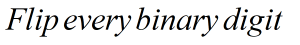Request a Tool

Ones Complement Calculator

Ones Complement Calculator is use to calculate ones complement of binary numbers.

Ones Complement
0

Formula• 100101 = 011010

Defination / Uses

Simply invert the supplied number to get the 1's complement of a binary number. The 1's complement of the binary number 110010, for example, is 001101.

The values obtained by inverting 0s and 1s and altering all the bits in the binary representation of the number are known as one's complement of binary numbers. It's largely utilised in computer science, where it has different impacts depending on how a computer represents numbers. As a result, the online one's complement converter can convert one's complement to decimal and decimal to one's complement, as well as binary, decimal, and one's complement to hexadecimal. A straightforward method exists for converting a binary number to its 1s complement. Simply exchange the provided integer with its counterpart to get the complement of a binary number.